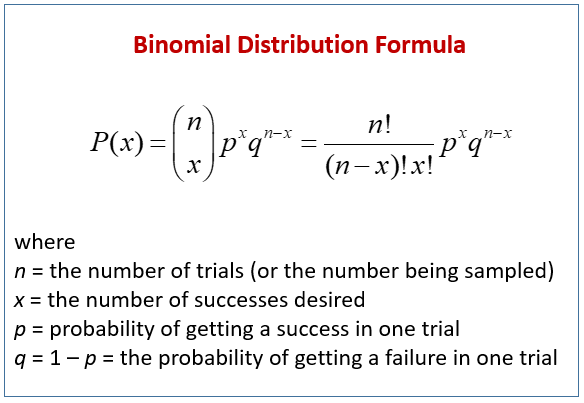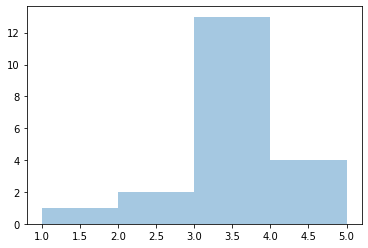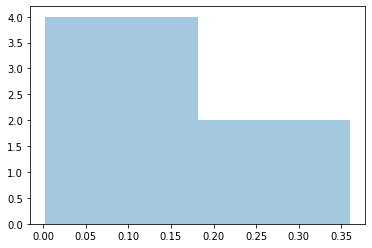# Binomial Distribution in Python

Manav Narula Jan 30, 2023 Jan 13, 2022

A binomial distribution is an essential concept of probability and statistics. It represents the actual outcomes of a given number of independent experiments when the probability of success and failure is known. It is possible only when exactly 2 outcomes are possible for a separate event, like a coin toss. Its mathematical formula is shown below.This tutorial will demonstrate how to create a binomial distribution in Python.

## Use the `numpy.random.binomial()` Function to Create a Binomial Distribution in Python

The `numpy` module can generate a series of random values in a `numpy` array. We can use the `numpy.random.binomial()` function to return a sample of this distribution.

We can specify the number of trials (`n`), probability of success (`p`), and size of the final output (`size`) as parameters in the function.

For example,

``````import numpy as np
a = np.random.binomial(n = 5, p = 0.7, size = 20)
print(a)
``````

Output:

``````[5 4 2 3 2 4 4 3 3 3 4 2 3 4 3 4 5 5 2 2]
``````

In the above example, each value represents the number of times an event occurs during `5` trials when the probability of success was `0.7`. This was repeated for a sample of size 20.

We can also plot this using the `seaborn.distplot()` function.

For example,

``````import matplotlib.pyplot as plt
import seaborn as sns
import numpy as np
a = np.random.binomial(n = 5, p = 0.7, size = 20)
sns.distplot(a, hist=True, kde=False)
plt.show()
``````

Output:## Use the `scipy.stats.binom.pmf()` Function to Create a Distribution of Binomial Probabilities in Python

The `scipy.stats.binom.pmf()` function returns the binomial probability for some given values. We can use it to create a distribution of binomial probabilities.

It is different from the previous distribution. We will loop over the number of successes desired to create this distribution.

For example,

``````from scipy.stats import binom
n = 5
p = 0.7
s = list(range(n + 1))
a = [binom.pmf(r, n, p) for r in s]
print(a)
sns.distplot(a, hist=True, kde=False)
plt.show()
``````

Output:Author: Manav Narula

Manav is a IT Professional who has a lot of experience as a core developer in many live projects. He is an avid learner who enjoys learning new things and sharing his findings whenever possible.